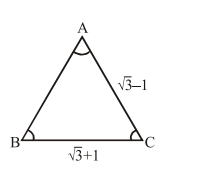# Solve this following

Question:

With the usual notation, in $\triangle A B C$, if

$\angle \mathrm{A}+\angle \mathrm{B}=120^{\circ}, \mathrm{a}=\sqrt{3}+1$ and $\mathrm{b}=\sqrt{3}-1$

then the ratio $\angle \mathrm{A}: \angle \mathrm{B}$, is :

1. $7: 1$

2. $5: 3$

3. $9: 7$

4. $3: 1$

Correct Option: 1

Solution:

$\mathrm{A}+\mathrm{B}=120^{\circ}$$\tan \frac{\mathrm{A}-\mathrm{B}}{2}=\frac{\mathrm{a}-\mathrm{b}}{\mathrm{a}+\mathrm{b}} \cot \left(\frac{\mathrm{C}}{2}\right)$

$=\frac{\sqrt{3}+1-\sqrt{3}+1}{2(\sqrt{3})} \cot \left(30^{\circ}\right)=\frac{1}{\sqrt{3}} \cdot \sqrt{3}=1$

$\frac{\mathrm{A}-\mathrm{B}}{2}=45^{\circ}$

\begin{aligned} \Rightarrow \mathrm{A}-\mathrm{B} &=90^{\circ} \\ \mathrm{A}+\mathrm{B} &=120^{\circ} \end{aligned}

$2 \mathrm{~A}=210^{\circ}$

$\mathrm{A}=105^{\circ}$

$\mathrm{B}=15^{\circ}$

$\therefore$ Option (1)